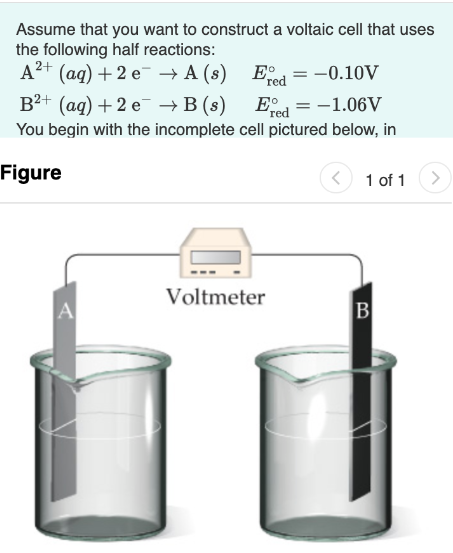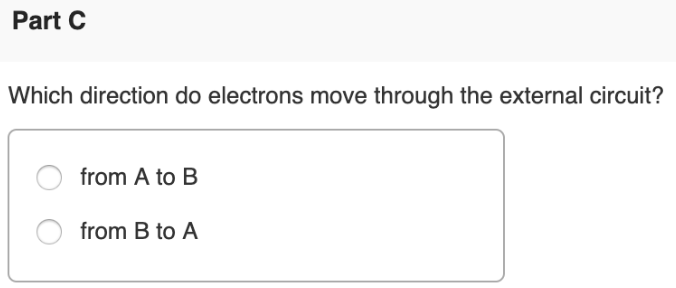# Assume that you want to construct a voltaic cell that uses the following half reactions: A^2+(aq) + 2 e^- → A (s) E°red = -0.10V B^2+(aq) + 2 e^- → B (s) E°red = -1.06V You begin with the incomplete cell pictured below, in Which direction do electrons move through the external circuit? a) from A to B b) from B to A